After all Covid-related hassle, a new micropost!

Do you know how to calculate the induced voltage – or coil fluxes, for that matter – from finite element analysis results?

Most of the time, nobody cares. After all, your software of choice is supposed to do that for you. But, it’s still worthwhile to educate oneself every now and then. Besides, it’s not that uncommon to get a bunch of raw data from a client, subcontractor, or a retired coworker, and having to figure out things yourself.

## First, some basics.

By definition, coil flux is the surface integral of the flux density, over a surface enclosed by the coil: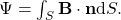Now, looks good on paper – at least to a high school physics student. In practice, there are a few complications

## Issues

First, coils are sadly not infinitely thin. Instead, they usually consist of several series-turns of smaller or larger conductors. Thus, the outermost turns actually enclose a larger surface than the innermost ones. For very large conductors, such as hairpins, the inner part of a single conductor may enclose a significantly smaller area than the outermost part.

The second issue is related to computational accuracy. Most magnetics problems – by far the most – are solved (under the software hood) with the vector potential (A) formulation, defined by: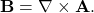Since the flux density is now a derivative of our primary quantity A, it is considerably less accurate. Meaning, if we use B directly, our results won’t be as reliable as possible.

## Solution in 2D

### Getting rid of derivatives

The solution to both of these results is relatively obvious in 2D. The only special trick we have to know is the Stokes theorem: After plugging in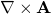in place of B, we can transform the above surface integral into a line integral without any derivatives: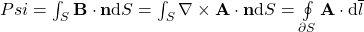.

In other words, we have replaced the surface integral by a line integral, across the surface boundary.

As this gets us rid of the derivative, we have solved the second issue.

### Accounting for coil size

The first issue – large area of each coil side – is also easy to solve in 2D. Remember, we are speaking about a 2D solution, with the field entirely in the xy-plane. This means that the vector potential is entirely z-directional, and that the end-winding fluxes contribute nothing to the computed flux.

Now, remember that we are speaking about a coil. The coil consists of the active part – two z-directional slot-bound coil-sides inside the motor core – plus then the end-winding. As the end-winding does not contribute to the computed flux in 2D, we are left with just the two coil-sides.

Therefore, the above line integral is actually simplified into a simple evaluation of the vector potential at the coil sides: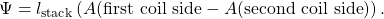The above expression still assumes the coil sides to be extremely thin. To account for the non-zero size, we can use a simple average: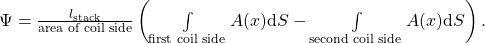In plain words, we get the flux linked by a single coil by calculating the average vector potential over each coil side, and then multiplying their difference by the problem length.

Check out EMDtool - Electric Motor Design toolbox for Matlab.

Need help with electric motor design or design software? Let's get in touch - satisfaction guaranteed!### 4 thoughts on “Computing Flux Linkages in 2D”

•Raghu Ramachandran says:

Nice post Antti! Does this mean though that in 3D, one would have to account for the end-winding and the end winding flux, and that the only reason the fields are confined to the x-y plane in 2D is because we only have conductors in the z-direction? (Of course it does!)

Also a sort of related question. In my FEM software, I am able to plot the “d-axis” and “q-axis” flux linkage for a motor. What is the physical meaning of this, i.e. does one count flux lines (in arbitrary units) in the d and q directions?

1.Antti (admin) says:

Thank you! That’s exactly what it means; 3D analysis also accounts for the end-winding flux, while 2D analysis is by definition confined to the 2D-plane (as though the problem were infinitely long in the z-direction).

The d- and q-axes, on the other hand, are more related to circuit analysis. The flux linkages are first computed for each phase, using e.g. the method in this post. So nothing special in this step.

Then, the three phase quantities are transformed with a linear transformation, into the d- and q-axis components and optionally a third 0-component.The transformation is defined so that a balanced sinusoidal 3-phase system gets transformed into constant d- and q-axis components. Furthermore, the d-axis is typically selected to coincide with the permanent magnet flux (or easy magnetization axis in case of synchronous reluctance machines).

Why we do this is due to two reasons at least. First of all, salient-pole machines are easier to analyze in the dq-frame, as we’re dealing with (almost) constant quantities. The second reason is related to the first: controllers are easier to implement in the dq-frame, as we’re controlling a DC-quantity rather than a constantly-changing sinusoid.

•Marcel says:

Hi Antti,
First of all, congratulations for your posts! You make them very interesting, clear and rich of good information.

When you said to “replaced the surface integral by a line integral, across the surface boundary”.
Which boundary we must consider for this line integral?

1.Antti (admin) says:

Ah, good question! The terms ‘boundary’ and ‘line’ actually, now that I think of it, only apply to 3D analysis. Consider a single-loop coil, made of thin wire. Then, the ‘surface’ in question would be some surface with the coil-wire as its boundary. The exact shape does not matter – it could be plane, or a colossal bubble – the flux linkage would still be the same, thanks to the fact that the magnetic field lines always for closed loops.

The line integral would then be along the wire itself.

Now, in 2D analysis, the coil would be assumed to be infinitely long in the z-direction, and the field to lie in the xy-plane only (Bz=0 everywhere). Here, the surface inside the coil would be seen as a line in the 2D plane. And the line integral along the wire would be reduced into a point evaluation multiplied by the length of the problem in the z-direction.